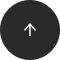#### Operators:

title: "How to Find the Average of a Numeric Column in SQL" database: ["SQL", "PostgreSQL", "MS SQL Server", "Oracle", "SQL Server", "MySQL", "SQLite"] tags: ["SQL", "PostgreSQL", "MS SQL Server", "Oracle", "SQL Server", "MySQL", "SQLite"] operators: AVG related_courses: - "sql-queries" - "ms-sql-basics" - "sql-practice-set" related_articles: - "introduction-using-aggregate-functions-joins" - "beginners-guide-sql-aggregate-functions" related_cookbooks: - "how-to-count-distinct-values-in-sql" - "how-to-order-by-count-in-sql" - "how-to-sum-values-of-a-column-in-sql" - "finding-maximum-values-in-rows" - "how-to-find-the-minimum-value-of-a-column-in-sql" - "how-to-round-numbers-in-sql" - "how-to-floor-numbers-in-sql" ---

#### Problem:

You’d like to calculate the average of numbers stored in a column.

#### Example:

Our database has a table named `sale` with data in the following columns: `id`, `city`, `year`, and `amount`.

idcityyearamount
1Los Angeles20172345.50
2Chicago20181345.46
3Annandale2016900.56
4Annandale201723230.22
5Los Angeles201812456.20
6Chicago201789000.40
7Annandale201821005.77
8Chicago20162300.89

Let’s calculate the average sales, regardless of city or year.

#### Solution:

```SELECT AVG(amount) as avg_amount
FROM sale;
```

Here’s the result:

avg_amount
19073.125000

#### Discussion:

If you’d like to calculate the average of numeric values stored in a column, you can do so using the `AVG()` aggregate function; it takes as its argument the name of the column whose average you want to calculate. If you haven’t specified any other columns in the `SELECT` clause, the average will be calculated for all records in the table.

Of course, since it’s an aggregate function, `AVG()` can also be used with groups. For example, if we’d like to find the average sale per city, we can write this query:

```SELECT city, AVG(amount) as avg_amount
FROM sale
GROUP BY city;
```

The average is calculated for each city:

cityamount
Los Angeles7400.850000
Chicago30882.250000
Annandale15045.516667

Additionally, if the average has to be rounded, you can pass the result of the `AVG()` function into `ROUND()`:

```SELECT city,
ROUND(AVG(amount), 2) as avg_amount
FROM sale
GROUP BY city;
```